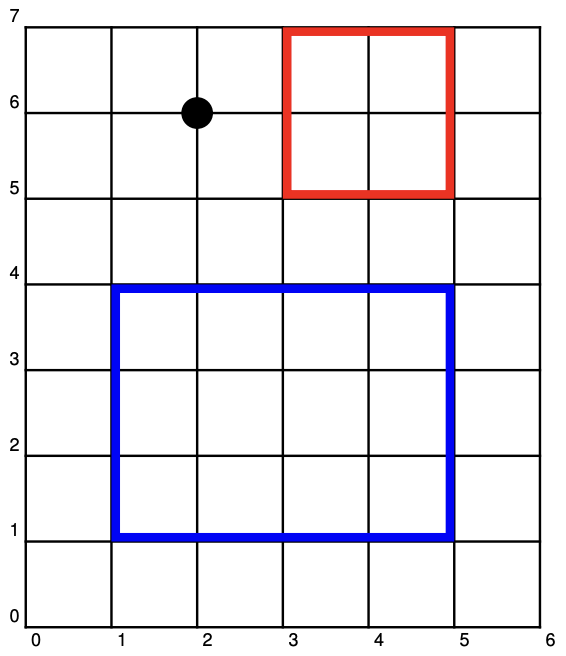시간 제한 메모리 제한 제출 정답 맞은 사람 정답 비율
1 초 512 MB 1 1 1 100.000%

## 문제

You are playing hide-and-go-seek in a forest with Belle.  The forest has one tree at each of the positive integer lattice points.  That is, there is a tree at every point $(x,y)$ where $x$ and $y$ are both positive integers.  You may consider each tree as a point.  A logging company has cut down all of the trees in some axis-aligned rectangle, including those on the boundary of the rectangle.

You are standing at $(0,0)$ and Belle is standing at $(x_b,y_b)$. You can see Belle if and only if there is no tree blocking your line of sight to Belle. If there is a tree at $(x_b,y_b)$, Belle will make it easier for you to find her by standing on the side of the tree facing your location.

For example, suppose that Belle is standing at $(2,6)$.  If the trees in the rectangle with corners at $(1,1)$ and $(5,4)$ are cut down (blue rectangle in figure), then you can see Belle.  However, if the rectangle was at $(3,5)$ and $(5,7)$ (red rectangle in figure), then the tree at $(1,3)$ would be in the way.Given the rectangle and Belle's location, can you see her?

## 입력

The first line of input contains two integer $x_b$ and $y_b$ ($1 \leq x_b,y_b \leq 10^{12}$), which are the coordinates that Belle is standing on.

The second line of input contains four integers $x_1$, $y_1$, $x_2$ and $y_2$ ($1 \leq x_1 \leq x_2 \leq 10^{12}$ and $1 \leq y_1 \leq y_2 \leq 10^{12}$), which specify two opposite corners of the rectangle at $(x_1, y_1)$ and $(x_2, y_2)$.

## 출력

If you can see Belle, display Yes.

Otherwise, display No and the coordinates of the closest tree that is blocking your view.

## 예제 입력 1

2 6
1 1 5 4


## 예제 출력 1

Yes


## 예제 입력 2

2 6
3 5 5 7


## 예제 출력 2

No
1 3


## 예제 입력 3

830844890448 39710592053
821266 42860 402207107926 423171345006


## 예제 출력 3

No
402207964848 19223704203